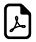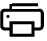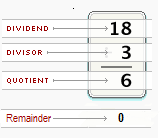# Terminology used in Division Problems## Terms Used In Division

Operations Vocabulary Explanation Example
Division Dividend The number being dividedDivisor Number that will divide the dividend exactly
Quotient The Result of Division
Remainder Number "left over" after dividing one Number by another

### Division Terms with Example:

20 ÷ 2 = 10 ,

Here, 20 is Dividend

2 is Divisor

10 is Quotient

0 is Remainder ( No left over after dividing)

###### These vocabulary of division is the basic things in general mathematics###See Also ►

Table of Squares, Cubes, Perfect Fourths & Fifths